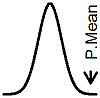P.Mean: A simple example of change of variable (created 2012-05-15). News: Sign up for "The Monthly Mean," the newsletter that dares to call itself average, www.pmean.com/news.I need to review some basic mathematical statistics in order to understand some of the Bayesian accrual models that I am developing. One of those things, that is actually quite easy, but I seem to have some trouble with is the method known as "change of variable." This is a method that allows you to characterize the probability distribution of a random variable that is transformed by a simple function. I wanted to illustrate how this works for a simple, but not trivial case, just to prove to myself that this works.

Assume that T is distributed as an exponential random variable with rate parameter lambda. The density function for T isNow consider the transformationWhat is the density function for P? It turns out that you can use brute force to solve this problem, or you can use a more elegant approach. Here's how you would use brute force. Notice first that the cumulative distribution function for the exponential distribution has a simple closed form solutionYou need to find the cumulative distribution function for P. Notice thatTo find the density function for P, simply take the derivative of the cumulative distribution function.This is a beta distribution with parameters lambda and 1.

Now how would you do this the elegant way? If T has density function f(t), the P=g(T) has density functionThis is a well known result and is a simple extension of the substitution of variables problem in Calculus. The term in absolute value is the Jacobian. This result only holds for monotone transformations, though it can be adapted for non-monotone transformations with a bit of work.

In our example,andso the density function for P iswhich simplifies toThis may seem like a trivial academic exercise, and it is. But there are practical problems that are similar in nature. In particular, I am interested in seeing how the prior distribution changes when you transform the Bayesian model. That requires the same change of variable technique as shown here.This page was written by Steve Simon and is licensed under the Creative Commons Attribution 3.0 United States License. Need more information? I have a page with general help resources. You can also browse for pages similar to this one at Statistical Theory.